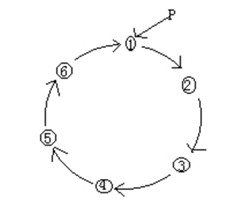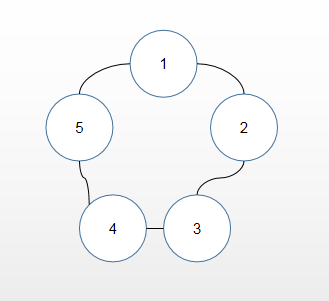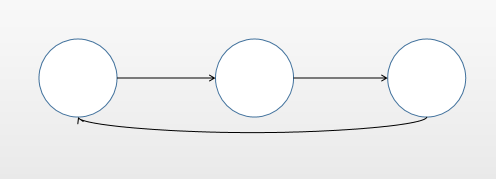# 图解Java数据结构之环形链表

##### 介绍##### 约瑟夫环问题1. 先创建第一个节点，让first指向该节点，并形成环状
2. 每创建一个新的节点就将该节点加入到已有的环形链表中

//创建一个环形的单向链表
// 创建一个first节点，当前没有编号
private Boy first = null;

// 添加节点，构建成一个环形链表
public void addBoy(int nums) {
// 对nums做一个校验
if (nums < 1) {
System.out.println("数据错误");
return;
}

// 定义辅助节点
Boy curBoy = null;

// 使用循环创建环形链表
for (int i = 1; i <= nums; i++) {
// 根据编号创建节点
Boy boy = new Boy(i);
// 如果是第一个节点
if (i == 1) {
first = boy;
first.setNext(first);
curBoy = first;// 让curBoy指向第一个节点，帮助构建链表
} else {
curBoy.setNext(boy);
boy.setNext(first);// 使其指向第一个节点，形成环状
curBoy = boy;// curBoy后移
}
}
}

// 遍历当前环形链表
public void list() {
// 判断链表是否空
if (first == null) {
System.out.println("链表为空");
return;
}
// 定义辅助节点
Boy curBoy = first;
while (true) {
System.out.println("节点编号:" + curBoy.getNo());
if (curBoy.getNext() == first) {
// 此时说明遍历完毕
break;
}
curBoy = curBoy.getNext();// curBoy后移
}
}
}

//创建一个Boy类，表示一个节点
class Boy {
private int no;// 编号
private Boy next;// 指向下一个节点

public Boy(int no) {
this.no = no;
}

public int getNo() {
return no;
}

public void setNo(int no) {
this.no = no;
}

public Boy getNext() {
return next;
}

public void setNext(Boy next) {
this.next = next;
}
}


public static void main(String[] args) {
}


节点编号:1



1. 先创建一个辅助节点helper，事先应该指向环形链表的最后一个节点
2. 报数前，先让first和helper移动k - 1次
3. 开始报数时，让first和helper节点同时移动，移动m - 1次
4. 此时可以将first指向的节点出圈
5. 如何出圈呢?
使first = first.next，即：将first节点往前移动一下
使helper.next = first，这样就跳过了要出圈的节点

	/**
* 根据用户的输入，计算出圈序列
*
* @param startNo  表示从第几个开始数
* @param countNum 表示数几下
* @param nums     表示一共有多少人
*/
public void countBoy(int startNo, int countNum, int nums) {
// 数据校验
if (first == null || startNo < 1 || startNo > nums) {
System.out.println("参数输入有误");
return;
}
// 定义辅助节点
Boy helper = first;
// helper事先应该指向环形链表的最后一个节点
while (true) {
if (helper.getNext() == first) {
break;
}
helper = helper.getNext();// helper后移
}
// 报数前，先让first和helper移动k - 1次
for (int j = 0; j < startNo - 1; j++) {
first = first.getNext();
helper = helper.getNext();
}
// 开始报数时，让first和helper节点同时移动，移动m - 1次
// 这里是一个循环的操作，直到圈中只有一个节点
while (true) {
if (helper == first) {
// 此时说明圈中只有一个人
break;
}
// 让first和helper同时移动countNum - 1次
for (int j = 0; j < countNum - 1; j++) {
first = first.getNext();
helper = helper.getNext();
}
// 此时first指向的节点就是要出圈的节点
System.out.println("节点" + first.getNo() + "出圈");
// 将该节点出圈
first = first.getNext();
helper.setNext(first);
}
System.out.println("最后留在圈中的节点编号:" + first.getNo());
}


	public static void main(String[] args) {

System.out.println("--------------");

// 测试出圈序列
}


节点编号:1

--------------



### 推荐阅读